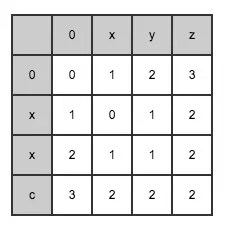# 尝试讲清楚编辑距离求解

1）删除h变成orse； 2）删除o变成rse； 3）增加o变成rose； 4）删除e变成ros

1）改h为r变成rorse； 2）删r变成rose； 3）删e变成ros

```def lev(a, b):
if len(a) == 0 or len(b) == 0:
return max(len(a),len(b))
if a == b:
return lev(a[1:],b[1:]) #首字符相等则跳过
DEL = lev(a[1:],b) # del a
REP = lev(a[1:],b[1:]) # set a = b, 问题转为求a[1:],b[1:]，原因同计算a的解释

• 增加：目标字符串减少一个字符；无论是加到头部还是加到尾部，加的都是目标字符串中的字符，相同的部分就不需要参与到后面的迭代了，毕竟我们要算的是最小操作数
• 删除：源字符串减少一个字符；这个不需要解释
• 替换：两个字符串都减少一个字符；同增加的道理一样，此时源字符串不仅不增加而且还可以减少一个

• 增加：a.append(b[-1])
• 删除：a.pop()
• 替换：a[-1] = b[-1]

lev(a, b[0:-1]); lev(a[0:-1],b);lev(a[0:-1],b[0:-1])

• if a[-1]==b[-1]: lev(a,b)  = lev(a[0:-1],b[0:-1])
• else: lev(a,b) = min(lev(a, b[0:-1])+1, lev(a[0:-1],b)+1, lev(a[0:-1],b[0:-1])+1)1）看下纵坐标i和横坐标j对应的两个字符a[j-1]和b[i-1]是否相同，如果相同转到第3步
2）计算ADD = dp[i][j-1]+1; DEL = dp[i-1][j]+1; REP = dp[i-1][j-1]+1，转到第4步
3）计算ADD = dp[i][j-1]+1; DEL = dp[i-1][j]+1; REP = dp[i-1][j-1]

```def minDistance(self, word1, word2):
l1 = len(word1)
l2 = len(word2)

if l1 == 0 or l2 == 0:
return max(l1, l2)

stat = [  * (l1+1) for i in xrange(l2+1) ]

# 直接给出所有原子问题的解
for i in xrange(l1+1):
stat[i] = i
for i in xrange(l2+1):
stat[i] = i

for i in range(1,l2+1):
for j in range(1,l1+1):
a = 0
if word1[j-1] == word2[i-1]:
a = stat[i-1][j-1]
else:
a = stat[i-1][j-1] + 1
# 每次扩展出的新问题都要把三种可能到达的操作考虑一遍
stat[i][j] = min(a,stat[i-1][j] + 1,stat[i][j-1] + 1)

return stat[l2][l1]
```